# Hodge structure

of weight(pure)
An object consisting of a latticein the real vector space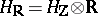and a decomposition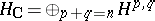of the complex vector space(a Hodge decomposition). Here the condition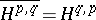must hold, where the bar denotes complex conjugation in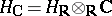. Another description of the Hodge decomposition consists in the specification of a decreasing filtration (a Hodge filtration)insuch that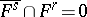for. Then the subspace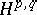can be recovered by the formula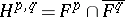.
An example is the Hodge structure in the-dimensional cohomology space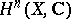of a compact Kähler manifold, which was first studied by W.V.D. Hodge (see ). In this case the subspacecan be described as the space of harmonic forms of type(cf. Harmonic form), or as the cohomology space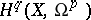of sheaves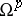of holomorphic differential forms . The Hodge filtration inarises from the filtration of the sheaf complex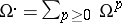, the-dimensional hypercohomology group of which is, by subcomplexes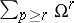.
A more general concept is that of a mixed Hodge structure. This is an object consisting of a lattice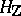in, an increasing filtration (a filtration of weights)in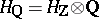and a decreasing filtration (a Hodge filtration)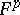in, such that on the space, the filtrationsanddetermine a pure Hodge structure of weight. The mixed Hodge structure in the cohomology spaces of a complex algebraic variety (not necessarily compact or smooth) is an analogue of the structure of the Galois module in the étale cohomology (cf. ). The Hodge structure has important applications in algebraic geometry (see Period mapping) and in the theory of singularities of smooth mappings (see ).